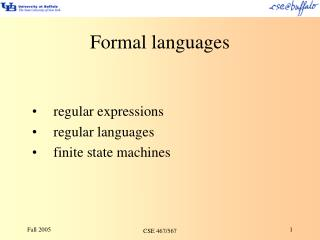# Formal languages - PowerPoint PPT PresentationDownload PresentationFormal languages

Download Presentation## Formal languages

- - - - - - - - - - - - - - - - - - - - - - - - - - - E N D - - - - - - - - - - - - - - - - - - - - - - - - - - -
##### Presentation Transcript

1. Formal languages • regular expressions • regular languages • finite state machines CSE 467/567

2. Formal languages A string is a (perhaps empty) sequence of symbols. e denotes the empty string. A language is a (perhaps empty) set of strings. Æ denotes the empty set. There are many different classes of languages. Main ones in Chomsky hierarchy are regular, context free, context sensitive and unrestricted. CSE 467/567

3. Sets and set operations Examples: Æ is the empty set, a set with no members {a, b, c} is a set with three members Operations: Suppose A={a, b, c} and B={1, 2}, then A·B={a1, a2, b1, b2, c1, c2} A´B={(a,1),(a,2),(b,1),(b,2),(c,1),(c,2)} AÈB={a, b, c, 1, 2} A*={e, a, b, c, aa, ab, ac, ba, bb, bc, aaa, aab, aac, …} CSE 467/567

4. Regular languages The class of regular languages over an alphabet å can be defined recursively: base case 1: Æ is a regular language base case 2: {e} is a regular language base case 3: for each symbol a in å, {a} is a regular language recursive cases: If S and T are regular languages, then so are: {st| s is in S and t is in T}, the concatenation of S and T {x| x is in S or x is in T}, the disjunction of S and T S*, the Kleene closure of S Nothing else is a regular language. CSE 467/567

5. Finite state automata Formally a 5-tuple (Q, å,q0,F,d) where Q is a finite set of states å is a finite input alphabet of symbols q0 Î Q is the initial state F Í Q is a set of final states d: Q´åQ CSE 467/567

6. Examples FSA (5-tuple and diagram) accepting each of the following (assume å={a,b,c,…z}): {a} {fred, wilma} {ball, bell, bill, boll, bull} {e, a} {e, a, b, c, aa, ab, ac, ba, bb, bc, ca, cb, cc, aaa, …} CSE 467/567

7. RE’s outside of Perl? Can always directly implement the DFA. • switch on state, switch on symbol • table-driven method (put d in an array) Example! CSE 467/567

8. Construction of FA Base cases • Æ is a regular language • {e} is a regular language • for each symbol a in å, {a} is a regular language Recursive cases If S and T are regular languages, then so are: • {st| s is in S and t is in T}, the concatenation of S and T • {x| x is in S or x is in T}, the disjunction of S and T • S*, the Kleene closure of S CSE 467/567

9. NFA vs. DFA Accept same set of languages. Simulation of NFA through search: depth-first (stack regime for next node) breadth-first (queue regime) Can mechanically convert NFA to DFA, with possible exponential increase in number of states. CSE 467/567